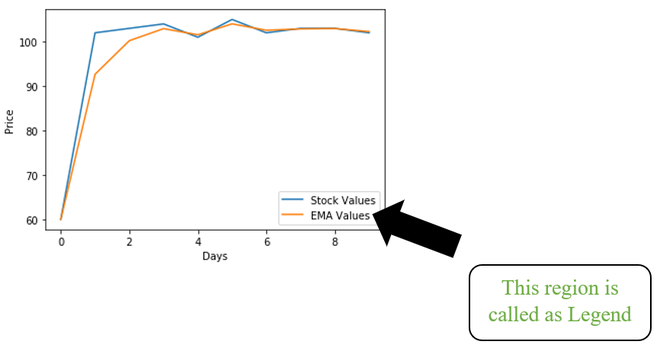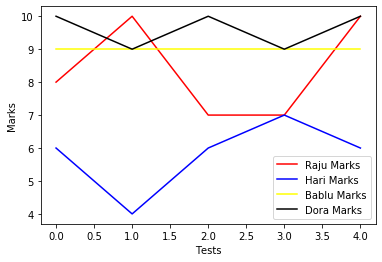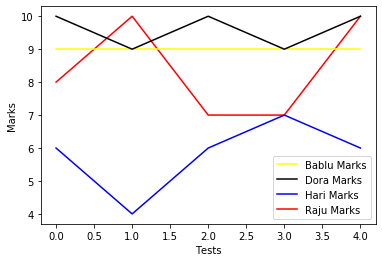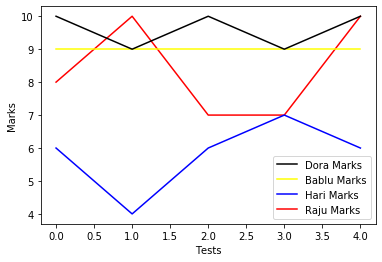GeeksforGeeks App
Open AppBrowser
Continue

# How to Change Order of Items in Matplotlib Legend?

Legend is a method present in the Matplotlib package which represents the area to describe the elements in a plot/graph.The order of labels in the legend region was based on the order we add the lines(data) to the plot.

Example:

Let us consider a DataFrame that stores student marks in weekly tests.

The above table stores the 4 student marks in 5 tests. Now plot the graph with legend using the above data.

## Python3

 `# import necessary packages``import` `pandas as pd``import` `matplotlib.pyplot as plt`` ` `# create a dataframe``Marks ``=` `pd.DataFrame({``'Raju'``: [``8``, ``10``, ``7``, ``7``, ``10``],``                      ``'Hari'``: [``6``, ``4``, ``6``, ``7``, ``6``],``                      ``'Bablu'``: [``9``, ``9``, ``9``, ``9``, ``9``],``                      ``'Dora'``: [``10``, ``9``, ``10``, ``9``, ``10``]})`` ` `# plot marks of each student``plt.plot(Marks[``'Raju'``], label``=``"Raju Marks"``, color``=``"Red"``)``plt.plot(Marks[``'Hari'``], label``=``"Hari Marks"``, color``=``"Blue"``)``plt.plot(Marks[``'Bablu'``], label``=``"Bablu Marks"``, color``=``"Yellow"``)``plt.plot(Marks[``'Dora'``], label``=``"Dora Marks"``, color``=``"Black"``)`` ` `# labelling the axes``plt.xlabel(``"Tests"``)``plt.ylabel(``"Marks"``)`` ` `# add legend to plot``plt.legend()``plt.show()`

Output## Change order of items in the legend

The above order of elements in the legend region can be changed by the gca method that uses another sub-method called get_legend_handles_labels method.

Syntax

handles, labels = plt.gca().get_legend_handles_labels()

These handles and labels lists are passed as parameters to legend method with order of indexes.

Consider the below example code for detailed understanding.

Example 1:

In this code, we used the same DataFrame we used in the above code. But used the above-specified methods to change the order of elements in the legend region.

## Python3

 `# import necessary packages``import` `pandas as pd``import` `matplotlib.pyplot as plt`` ` `# create a dataframe``Marks ``=` `pd.DataFrame({``'Raju'``: [``8``, ``10``, ``7``, ``7``, ``10``],``                      ``'Hari'``: [``6``, ``4``, ``6``, ``7``, ``6``],``                      ``'Bablu'``: [``9``, ``9``, ``9``, ``9``, ``9``],``                      ``'Dora'``: [``10``, ``9``, ``10``, ``9``, ``10``]})`` ` `# plot marks of each student``plt.plot(Marks[``'Raju'``], label``=``"Raju Marks"``, color``=``"Red"``)``plt.plot(Marks[``'Hari'``], label``=``"Hari Marks"``, color``=``"Blue"``)``plt.plot(Marks[``'Bablu'``], label``=``"Bablu Marks"``, color``=``"Yellow"``)``plt.plot(Marks[``'Dora'``], label``=``"Dora Marks"``, color``=``"Black"``)`` ` `# labelling the axes``plt.xlabel(``"Tests"``)``plt.ylabel(``"Marks"``)`` ` `# reordering the labels``handles, labels ``=` `plt.gca().get_legend_handles_labels()`` ` `# specify order``order ``=` `[``2``, ``3``, ``1``, ``0``]`` ` `# pass handle & labels lists along with order as below``plt.legend([handles[i] ``for` `i ``in` `order], [labels[i] ``for` `i ``in` `order])``plt.show()`

OutputExample 2:

Below is the implementation of plotting a graph that represents student marks along with legend with specified order in legend region.

## Python3

 `# import necessary packages``import` `pandas as pd``import` `matplotlib.pyplot as plt`` ` `# create a dataframe``Marks ``=` `pd.DataFrame({``'Raju'``: [``8``, ``10``, ``7``, ``7``, ``10``],``                      ``'Hari'``: [``6``, ``4``, ``6``, ``7``, ``6``],``                      ``'Bablu'``: [``9``, ``9``, ``9``, ``9``, ``9``],``                      ``'Dora'``: [``10``, ``9``, ``10``, ``9``, ``10``]})`` ` `# plot marks of each student``plt.plot(Marks[``'Raju'``], label``=``"Raju Marks"``, color``=``"Red"``)``plt.plot(Marks[``'Hari'``], label``=``"Hari Marks"``, color``=``"Blue"``)``plt.plot(Marks[``'Bablu'``], label``=``"Bablu Marks"``, color``=``"Yellow"``)``plt.plot(Marks[``'Dora'``], label``=``"Dora Marks"``, color``=``"Black"``)`` ` `# labelling the axes``plt.xlabel(``"Tests"``)``plt.ylabel(``"Marks"``)`` ` `# reordering the labels``handles, labels ``=` `plt.gca().get_legend_handles_labels()`` ` `# specify order``order ``=` `[``3``, ``2``, ``1``, ``0``]`` ` `# pass handle & labels lists along with order as below``plt.legend([handles[i] ``for` `i ``in` `order], [labels[i] ``for` `i ``in` `order])``plt.show()`

OutputMy Personal Notes arrow_drop_up